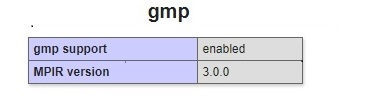# PHP - gmp Functions

## What is GMP?

GMP stands for GNU Multiple Precision Arithmetic Library (GMP).

GMP is a library supported in PHP that allows you to do mathematical operations on signed integers, rational numbers, and floating point numbers.GMP has a rich collection of functions that helps to perform complex mathematical operations on big numbers. GMP is said to be faster in computing the final result on very huge numbers.

GMP is very useful in applications like cyptography, internet security, and also in softwares that deal with arithmetic operations.

## GMP installation in PHP

GMP module is by default added to PHP. You can activate the same by removing the (;) ;extension=gmp added at the start of the extension in php.ini. After that restart apache and to confirm if the changes are reflecting.Save below code as .php and execute the same in the browser.

```<?php
phpinfo();
?>
```

You should see the GMP enabled in the browser as shown below −## GMP functions

Following table lists down all the functions related to PHP GMP functions. Here column version indicates the earliest version of PHP that supports the function.

Sr.No Function & Description Version
1 gmp_abs()

Returns the absolute value

5.0.0

Returns the sum of two numbers

5.0.0
3 gmp_and()

Returns the bitwise AND comparision of two numbers

5.0.0
4 gmp_binomial()

Calculates the binomial coefficient

7.3.0
5 gmp_clrbit()

Clears the bit

5.0.0
6 gmp_cmp()

Compares two GMP numbers

5.0.0
7 gmp_com()

Calculates one's complement

5.0.0
8 gmp_div_q()

Divides the given numbers

5.0.0
9 gmp_div_qr()

Divides the given numbers and returns quotient and remainder

5.0.0
10 gmp_div_r()

Divides the given numbers and returns the remainder

5.0.0
11 gmp_div()

Divides the given numbers

5.0.0
12 gmp_divexact()

Gives exact division of given numbers

5.0.0
13 gmp_export()

Exports a binary string

5.6.1
14 gmp_fact()

Calculates factorial of given number

5.0.0
15 gmp_gcd()

Calculates GCD of given numbers

5.0.0
16 gmp_gcdext()

Calculates the GCD and multipliers for the given numbers

5.0.0
17 gmp_hamdist()

Calculates the hamming distance between two given numbers

5.0.0
18 gmp_import()

Imports from a binary string

5.6.1
19 gmp_init()

Creates a GMP number

5.0.0
20 gmp_intval()

Converts GMP number to an integer

5.0.0
21 gmp_invert()

Returns the modular inverse of given GMP numbers

5.0.0
22 gmp_jacobi()

Calculates the jacobi symbol

5.0.0
23 gmp_kronecker()

Calculates the Kronecker symbol

7.3.0
24 gmp_lcm()

Calculates LCM of the given GMP numbers

7.3.0
25 gmp_legendre()

Calculates the Legendre symbol

5.0.0
26 gmp_mod()

Returns the modulus

5.0.0
27 gmp_mul()

Multiplies the GMP numbers

5.0.0
28 gmp_neg()

Returns the negative value of the given number

5.0.0
29 gmp_​nextprime()

Helps to find the next prime number

5.2.0
30 gmp_or()

Calculates the bitwise OR of the given numbers

5.0.0
31 gmp_​perfect_​power()

Checks if the GMP number is a perfect power

7.3.0
32 gmp_perfect_square()

Checks if the GMP number is a perfect square

5.0.0
33 gmp_popcount()

Calculates the population count

5.0.0
34 gmp_pow()

Returns the result for the given number raise to the power

5.0.0
35 gmp_powm()

Returns the new number with the given number raised to the power along with modulo

5.0.0
36 gmp_prob_prime()

Checks if the GMP number is prime number or not

5.0.0
37 gmp_random_bits()

Returns random number

5.6.3
38 gmp_random_range()

Returns random number between the min and max numbers given

5.6.3
39 gmp_random_seed()

Sets the seed to generate the randon number

7.0.0
40 gmp_random()

Returns random number.

5.0.0
41 gmp_root()

Returns the integer value for the root

5.6.0
42 gmp_rootrem()

Returns the integer value and remainder from the nth root

5.6.0
43 gmp_scan0()

Scans the 0's in the given number

5.0.0
44 gmp_scan1()

Scans the 1's in the given number

5.0.0
45 gmp_setbit()

Sets the bit index for the given GMP number

5.0.0
46 gmp_sign()

Returns the sign of the given number

5.0.0
47 gmp_sqrt()

Calculates the square root

5.0.0
48 gmp_sqrtrem()

Calculates the square root with remainder

5.0.0
49 gmp_strval()

Converts given GMP number to a string

5.0.0
50 gmp_sub()

Returns the difference between two given numbers

5.0.0
51 gmp_testbit()

Tests if the bit is set for the given index

5.3.0
52 gmp_xor()

Calculates the bitwise XOR of the given numbers

5.0.0
php_function_reference.htm